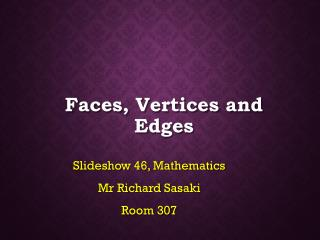Download PresentationFaces, Vertices and Edges

# Faces, Vertices and Edges - PowerPoint PPT Presentation

Faces, Vertices and Edges. Slideshow 46, Mathematics Mr Richard Sasaki Room 307. Objectives. Recall names of some common 3d Shapes Recall the meaning of face, vertex and edge Be able to count the number of faces, vertices and edges for a polyhedron and use the formula that relates them.I am the owner, or an agent authorized to act on behalf of the owner, of the copyrighted work described.
Download Presentation## Faces, Vertices and Edges

An Image/Link below is provided (as is) to download presentation

Download Policy: Content on the Website is provided to you AS IS for your information and personal use and may not be sold / licensed / shared on other websites without getting consent from its author.While downloading, if for some reason you are not able to download a presentation, the publisher may have deleted the file from their server.

- - - - - - - - - - - - - - - - - - - - - - - - - - E N D - - - - - - - - - - - - - - - - - - - - - - - - - -
Presentation Transcript
1. Faces, Vertices and Edges Slideshow 46, Mathematics Mr Richard Sasaki Room 307

2. Objectives Recall names of some common 3d Shapes Recall the meaning of face, vertex and edge Be able to count the number of faces, vertices and edges for a polyhedron and use the formula that relates them

3. Some Simple 3-D Shapes Cylinder Cone Sphere Square-based pyramid Hemisphere

4. Platonic solids / conVEX regular polyhedrons Cube Tetrahedron Octahedron Dodecahedron Icosahedron

5. Prisms Triangular Prism Cuboid Hexagonal Prism Pentagonal Prism Octagonal Prism Decagonal Prism Dodecagonal Prism Heptagonal Prism

6. FaceS We know a face is a flat finite surface on a shape. What is a face? This makes the term easy to use for polyhedra and 2D shapes. But for a sphere…does it have zero faces?

7. Faces There is some disagreement about curved faces. 3D shapes that aren’t polyhedra don’t follow the laws and cause problems. But for us, a face is defined as a finite flat surface so we will use that definition and think of a sphere as having zero faces.

8. Vertices and edges Vertex - A point (with zero size) where two or more edges meet. Edge - Defining vertex and edge is a little easier. A line segment dividing two faces. Note: An edge may not always be connected with two vertices. The hemisphere and cone are examples of this.

9. 12 8 6 5 9 6 4 4 F+V-E=2 6 Edges can be curved (or meet themselves). Octahedron 6 30 Icosahedron 20 Decagonal Prism, 12 faces, 30 edges

10. F + V – E = 2(Euler’s formula) Let’s try a hemisphere. It has face(s). 1 Can you think of a 3D shape where this formula doesn’t work? It has vertex / vertices. 0 1 It has edge(s). Let’s substitute these into the equation. F + V – E = 2 = 0 1 + 0 – 1 ???

11. F + V – E = ???(Euler’s formula) F + V – E = 2 only works perfectly for shapes that are . convex polyhedra For 3D non-polyhedra, F + V – E may equal 0, 1 or 2 (and sometimes other numbers). This value, we call χ (read as chi.)

12. 10 15 2 0 0 0 0? 1 1 1 1 7 + 6 – 12 = 1 F + V – E = 6 + 8 – 12 = 2 7 F + V – E = 8 + 6 – 12 = 2 F + 12 – 24 = -2, F = 10 F + V – E = 11 + 18 – 27 = 2 2 + 0 – 2 = 0